# Simplification Problems: Practice Questions with Tricks for Bank & Other Exams

## Simplification Problems:

Simplification basics and tricks are available here. For all competitive exams, candidates can use these Most Important Simplification problems for practice. Tricks given here will be helpful to solve the questions very quickly and score good marks. Simplification sums are the most expected topics in all Competitive exams in the quantitative aptitude section and it is one of the Important Topics which help to Increase Your Score. So, practice more simplification for bank exam and other competitive exams. The simplification for bank PO exams will be slightly tricky and time-consuming. So, take more Simplification practice questions and boost your overall score. Also, take more Simplification quiz practice by which you can gain the ability to solve the questions quickly. Below we have given the List of Most Expected Types of Simplification Questions along with the Simplification Tricks.

#### What is Simplification?

Mathematics is a Complex Web. If you want to know the answer for How do I make this conclusion? Read this article then it will be so simple. Simplification will be an important topic on all competitive exams like SBI PO, SBI Clerk, IBPS PO, IBPS Clerk, SSC CGL, SSC CHSL, and others. Simplification means finding the solution from the complex calculation. So understanding the significance of simplification will enhance your overall mental prowess. In this article, you can get Tricks of Simplification, Techniques, and more questions to practice for Simplification.

## Types of Simplification Sums:

There are two possible ways to ask a question that is related to simplification. Simplification questions will take place in all important competitive exams because we can check the individual analytical thinking through the simplification.

• Finding the missing number from the calculation will be the First type of simplification. In this type to get a solution to approximate the given number or we can simplify using the basic operations.
• The second method will understand the relation between the numbers and simplify the numbers using the rules of simplification.

## Examples with an explanation for Simplification Problems:

1). (10.8×16×12)  + (3.6×56×9.2)   =?

1. 3941.35
2. 3966.89
3. 3928.32
4. 3649.19
5. 3645.19
Ans: c
Solution: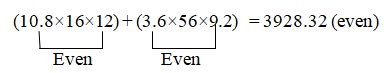Even + Even should be always Even. So the unit digit should be in even.
Note: All numbers should be Even.
2). 12.3% of 203 + 23% of  1110 =?
1. 270.269
2. 240.269
3. 280.269
4. 290.269
5. None of these
Ans :c
Solution: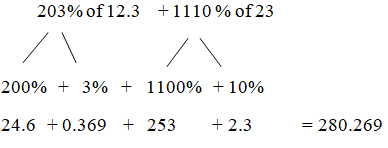Simplification Tricks :      A% of B = B % of A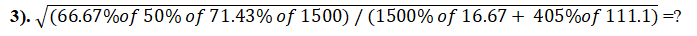1. 3/4
2. 5/7
3. 3/7
4. 4/7
5. None of these

Ans: b
Solution: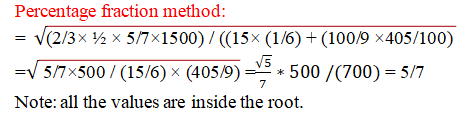4). 3545 ×14.75 – 2325 ×15.25 =?

1. 15369.5
2. 16832.5
3. 11546.5
4. 12475.5
5. None of these

Ans: b
Solution:
1220×15 – 5870 ×0.25 = 16832.5
(15+0.25=15.25; 15-0.25=14.75) (a-b , a+b)
Here,
3545+2325=5870 (x+y)
3545– 2325 =1220 (x-y)

5). 27% of 250 +22% of 920 +21% of 660=?

1. 419.5
2. 423.5
3. 408.5
4. 415.5
5. None of these

Ans: c
Solution:
Common percentage method:
(250+920+660) =1830
Here 20% is common;
20% of 1830 =366
Further,
1st part (20+7) = 7% of 250 =17.5
2nd part (20+2) = 2% of 920 =18.4
3rd part (20+1) = 1% 660 = 6.6
366+17.5+18.4+6.6 = 408.5

6). 212 +172 -132 = ?

1. 412
2. 512
3. 561
4. 461
5. None of these

Ans: c
Solution:
Method:
212 +(172 -132) = 212 +((17+13)(17-13)) = 441 +(30×4) =561
Note: (A2 – B2) = (a+b) (a-b)

7). (22×28)  +  (38×42) = ?

1. 2122
2. 2212
3. 2132
4. 2213
5. None of these

Ans: B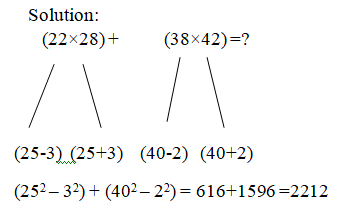Simplification Tricks:  If any two numbers in multiplication with the common base number, we can use this method

8). 12.75% of 464 =?

1. 59.96
2. 57.16
3. 51.86
4. 59.16
5. None of these

Ans: D
Solution:
(12.75×4) of (464/4) = 51% of 116 =59.16
Simplification Tricks: If any fractional number ends with 5, we can convert that into a whole number by this method.

9). 8.2% of 780 +x= 12.9% of 1310

1. 107.26
2. 106.53
3. 105.03
4. 103.56
5. None of these

Ans: c
Solution:
82×78/100 + X =129×131 /100
(130-1)(130+1) /100 – (80+2)(80-2) /100 =X
X=105.03
10). 0.875 of 848  + 0.625 of 624= ?

1. 1132
2. 1325
3. 1236
4. 1250
5. None of these

Ans: A
Solution:
87.5% of 848 + 62.5% of 624
(7/8 ×848) +  5/8 ×624 = 742 +390 =1132
Simplification Tricks: 87.5% = (100-12.5)%
= (1-1/8) =7/8

Simplification Tricks and Techniques:

Simplification -large, complex numerical expression into a simpler form by performing various mathematical operations, in accordance with the BODMAS rule.B  àStands for bracket and operation of brackets in the order ( ),( ),{ }and[ ]
OàStands for ‘of ‘(usage is x)
DàStands for division (/)
MàStands for multiplication (x)
à Stands for subtraction (-)

## Simplification Tricks –Easiest way to choose simplification questions:

STEP 1: Know about BODMAS Rule. Following are the list of priority given for brackets and signs.
STEP 2: If an expression Contains brackets, the expression within the brackets should be simplified first.
STEP 3: If an expression contains ‘Of’, multiplication, division, addition, and subtraction, then of should be performed first then followed by multiplication or division.
Proceeding from left to right, addition, and subtraction are carried out in the order in which the sign of addition and subtraction are given.
If an expression contains ‘Of’ and Division – always do ‘Of’ and then do division
STEP 4: If expression involves all four operations, then multiplication and division are carried out first in the order in which they are given from left to right. The same rules are carried out for addition and subtraction.

### Simplification Tricks – Squares(12 to 302):

·        1– 1
·        2– 4
·        3– 9
·        4– 16
·        5– 25
·        62 – 36
·        72– 49
·        82 – 64
·        92– 81
·        102-100
·        11-121
·        122-144
·        132 – 169
·        142– 196
·        152 – 225
·        162– 256
·        172 – 289
·        182– 324
·        192 – 361
·        202 – 400
·        212 – 441
·        222– 484
·        232 – 529
·        242– 576
·        252 – 625
·        262 – 676
·        272 – 729
·        282 – 784
·        292 – 841
·        302 – 900

### Simplification Tricks – Cubes (13to 153):

·        13 – 1
·        23– 8
·        33 – 27
·        43 – 64
·        53 – 125
·        63 – 216
·        73 – 343
·        83 – 512
·        93 – 729
·        103 – 1000
·        113 – 1331
·        123 – 1728
·        133 – 2197
·        143 – 2744
·        153 – 3375

Example 1: 212 / 49 × 6

Solution: From the above question if we know the square value of 212, then this question will be easily solved
STEP 1:212= 441
STEP 2:441/49= 9
STEP 3:9×6 = 54
STEP 4:Hence the answer for the above series is 54

### REMEMBER FREQUENTLY ASKED FRACTION VALUES (Simplification Tricks)

·        5% = 0.05
·        6 ¼ % = 0.0625
·        10% = 0.1
·        12 ½ = 0.125
·        16 × (2/3)% = 0.166
·        20 % = 0.2
·        25 % = 0.25
·        33 × (1/3)%= 0.33
·        40 % = 0.4
·        50% = 0.5
·        60% = 0.6
·        66 × (2/3) =0.66
·        75 %= 0.75
·        80 %= 0.8
·        90 % = 0.9
·        100% = 1
·        125 % = 1.25
·        150% = 1.5
·        200 % = 2
·        250 % =2.5
Example 2): 60% of 250 +25% of 600
STEP 1: Know the values of 60% =0.6 and 25 % = 0.25
STEP 2: Now directly multiply 0.6×250 + 0.25×600
STEP 3:0.6×250= 150
0.25×600=150
STEP 4: 150+ 150 = 300
STEP 5:Hence the answer for the above series is 300

Example 3): Solve mixed fraction – Multiplication
EXAMPLE 3: 2×(3/5) × 8×(1/3) + 7 ½ × 2×(2/3)
STEP 1:2×(3/5) × 8×(1/3) = (13/5) × (25/3) = 65/3
STEP 2:  + 7 ½ ×2×(2/3)= 43/6 × 12/5 = 86/5
STEP 3:65/3 + 86/5 = 38×(15/13)
STEP 4:hence the answer for the above series is38×(15/13)
Example 4): Solve Mixed Fraction addition
Example 4:19×(3/5) + 23×(2/3) – 24×(1/5)
STEP 1:Take all the whole number outside the bracket i.e. 19+23 -24 = 18
STEP 2:Add fractions within bracket 18×[(3/5) + (2/3) – (1/5)] = 18(16/15)
STEP 3:  Hence the answer for the above series is18(16/15)

Example 5): (?)2+18×12= 62×5×2
STEP 1:Multiply 18 × 12 = 216
STEP 2:Square of 6 = 36
STEP 3:Multiply 36 ×5×2= 360
STEP 4:(X)+216 = 360
STEP 5:(X)2  = 360-216 = 144
STEP 6:Therefore X = 12